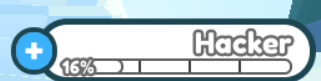# How Would I Have Level Names?Let’s Say I’m Level 100 So I Want The Tag To Be “HACKERMAN”
so every level has a special name.

2 Likes

Here’s how I would go about making such a system.

``````local levels = {1, 100} -- table containing all level milestones, must be in order from least-greatest
local tags = { = "Noob",  = "HACKERMAN"} -- dictionary mapping level milestones to tags

local function onLevel(level)
local rank
for i, minLevel in ipairs(levels) do
if level < minLevel then
-- the player is not high enough to reach this rank
-- thus they must fall in the previous category
rank = levels[i - 1]
break
end
end

-- if the player is the max rank they'll reach the end of loop
-- having never set the rank because their level will never be less
-- than any level in the table
if not rank then
rank = levels[#levels] -- set the rank to the last value in the table
end

return tags[rank]
end
``````
1 Like

I will supose that you have some knowledge on Datastore and Tables, but this is quite simple:

This is just an example

``````
local LevelsTable = {

 = "Starter",
 = "Beginner",
 = "Normal",
 = "Normal",
 = "Normal",
 = "Normal",
 = "Normal",
 = "Normal",
 = "Normal",
 = "Normal",

--Etc
}
local player = game.Players.LocalPlayer

local Reference = Instance.new("IntValue", player) <<<< Like this
Reference.Name = "Level" -- The name of IntValue
Reference.Value = 0 -- default value? i don't know how your system is

local PlrLevel = player.Level -- this is a "IntValue" inside the player

PlrLevel:GetPropertyChangedSignal("Value"):Connect(function()

print("Level Changed to: " .. PlrLevel .Value)

if PlrLevel .Value == LevelTable[PlrLevel.Value] then
--Reference of TextLabel.Text here

--TextLabel.Text = LevelsTemplate[PlrLevel.Value] -- The text will be what [LEVEL] was referenced
-- works with any Level

--LevelsTemplate could be a Module, where you would store all Level and stuff of the player
--LevelsTemplate is a table, so when the function is ran, i will get the matching [Player Level]
--then it will change the [Text] to what you wanted to be

else

end
end)
``````